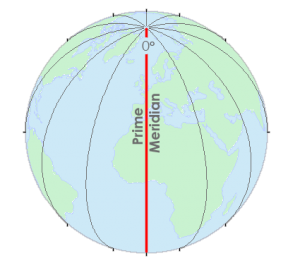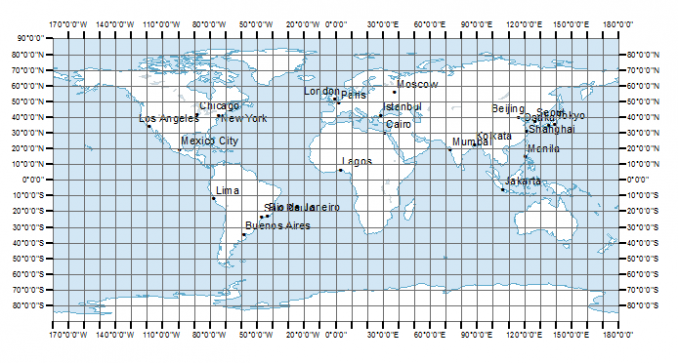# Greenwich Meridian (Prime Meridian)

Longitude lines like the Greenwich Meridian run north-south.

They converge at the poles. And X-coordinates are between -180 and +180, which are called longitudes.

But there’s a special longitudinal line that is the zero from which we measure east and west.

This is called the Greenwich Meridian (or prime meridian)

### Greenwich Meridian (Prime Meridian)

Using geographic coordinates, we can give a position to anything on Earth.

But how do we know where the longitude line of zero degrees begins?

In 1851, Sir George Airy established Royal Observatory in Greenwich, England to be the zero degree line of longitude. This is why prime meridian is also known as the Greenwich Meridian.In a geographical coordinate system, the Greenwich Meridian (or prime meridian) is the 0° line of longitude from which we measure 180° to the west and 180° to the east.

Most coordinate systems define a zero line at the equator that runs east-west. In a geographical coordinate system, the equator is a line of latitude defined to be 0°. Latitude ranges from +90° in the north direction to -90° in the south.### Geographic Coordinate Systems (GCS)

Latitude and longitude positions in a Geographic Coordinate Systems are based on spheroid or ellipsoid surfaces that approximate the surface of the earth.

All coordinates are referenced to a datum. A datum describes the shape of the Earth in mathematical terms. A datum defines the radius, inverse flattening, semi-major axis and semi-minor axis for an ellipsoid.

Longitudes:
X-coordinates are between -180 and +180, which are called longitudes.

Latitudes:
Y-values are between -90 and +90 degrees, which are lines of latitudes.

Coordinates are pairs (X, Y) or triplets (X, Y, Z) of values that are used to represent points and features on a two and three-dimensional space.

The X-value represents the horizontal position and Y-value represents the vertical position. The Z-value generally refers to the elevation at that point location.

### To Recap

The 0 degree line of longitude that passes through the Royal Observatory in Greenwich, England is called the Greenwich Meridian.

It’s also called the Prime Meridian.

This line is the starting point for longitudinal lines that run north-south and converge at the poles.

The Greenwich Meridian (or prime meridian) is a 0° line of longitude from which we measure 180° to the west and 180° to the east. These measurements are the basis of our geographic reference grid.